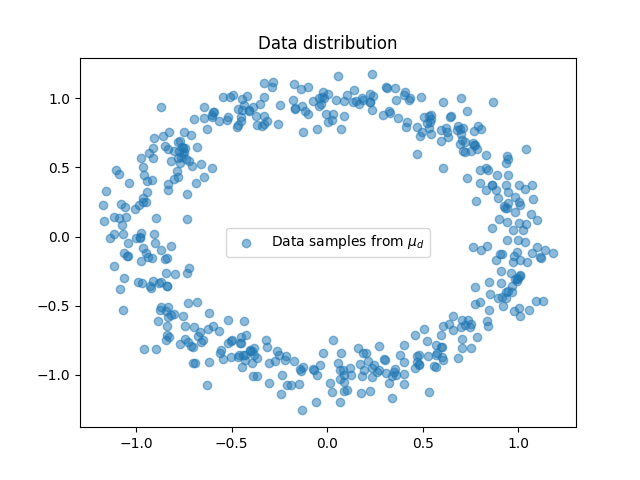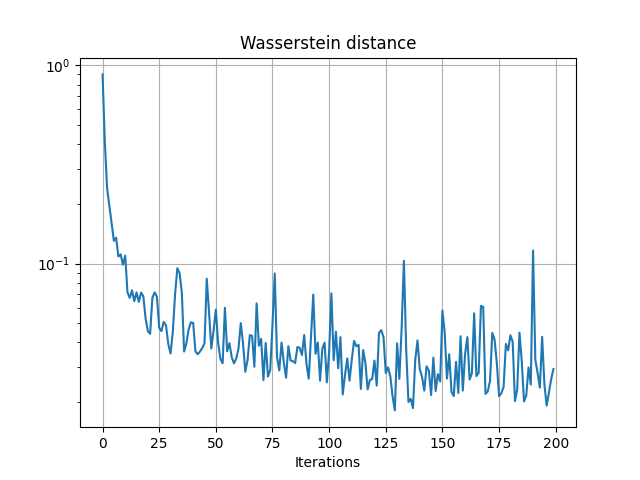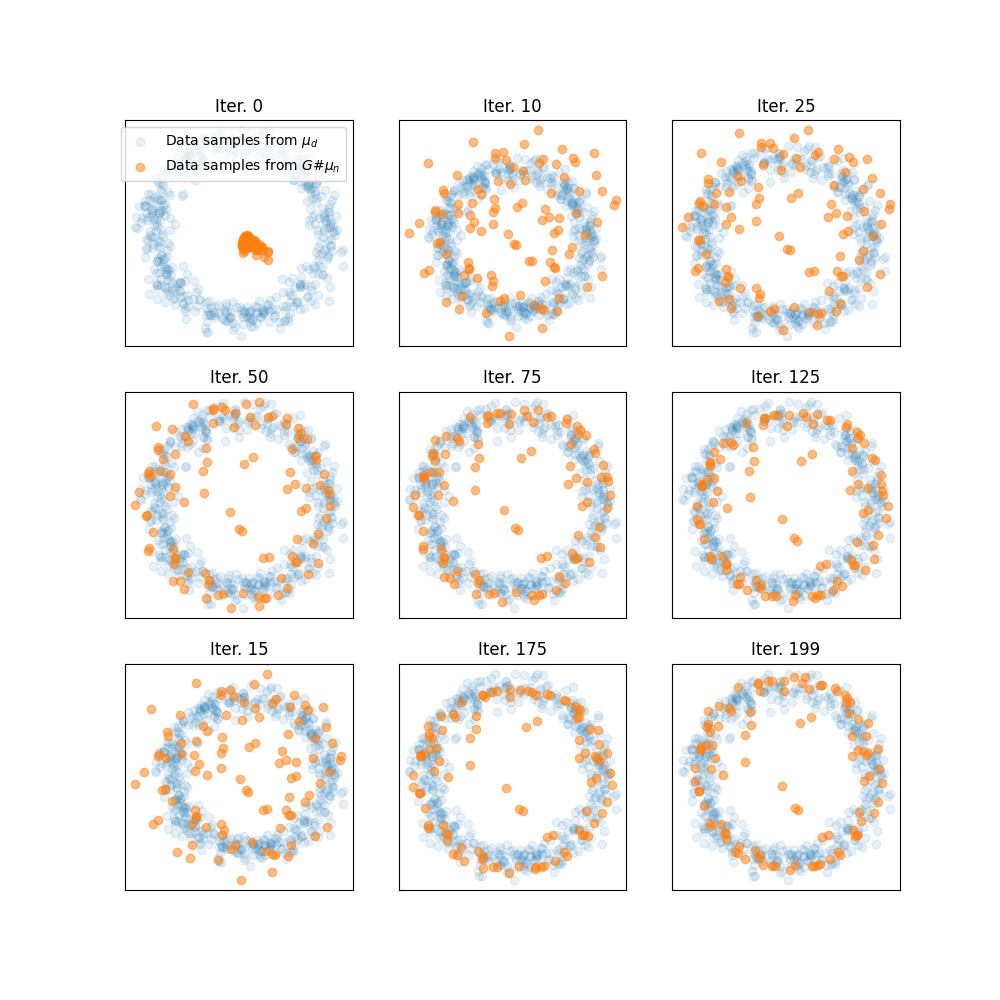# Wasserstein 2 Minibatch GAN with PyTorch

In this example we train a Wasserstein GAN using Wasserstein 2 on minibatches as a distribution fitting term.

We want to train a generator $$G_\theta$$ that generates realistic data from random noise drawn form a Gaussian $$\mu_n$$ distribution so that the data is indistinguishable from true data in the data distribution $$\mu_d$$. To this end Wasserstein GAN [Arjovsky2017] aim at optimizing the parameters $$\theta$$ of the generator with the following optimization problem:

$\min_{\theta} W(\mu_d,G_\theta\#\mu_n)$

In practice we do not have access to the full distribution $$\mu_d$$ but samples and we cannot compute the Wasserstein distance for lare dataset. [Arjovsky2017] proposed to approximate the dual potential of Wasserstein 1 with a neural network recovering an optimization problem similar to GAN. In this example we will optimize the expectation of the Wasserstein distance over minibatches at each iterations as proposed in [Genevay2018]. Optimizing the Minibatches of the Wasserstein distance has been studied in[Fatras2019].

[Arjovsky2017] Arjovsky, M., Chintala, S., & Bottou, L. (2017, July). Wasserstein generative adversarial networks. In International conference on machine learning (pp. 214-223). PMLR.

[Genevay2018] Genevay, Aude, Gabriel Peyré, and Marco Cuturi. “Learning generative models with sinkhorn divergences.” International Conference on Artificial Intelligence and Statistics. PMLR, 2018.

[Fatras2019] Fatras, K., Zine, Y., Flamary, R., Gribonval, R., & Courty, N. (2020, June). Learning with minibatch Wasserstein: asymptotic and gradient properties. In the 23nd International Conference on Artificial Intelligence and Statistics (Vol. 108).

# Author: Remi Flamary <remi.flamary@polytechnique.edu>
#

# sphinx_gallery_thumbnail_number = 3

import numpy as np
import matplotlib.pyplot as pl
import matplotlib.animation as animation
import torch
from torch import nn
import ot


## Data generation

torch.manual_seed(1)
sigma = 0.1
n_dims = 2
n_features = 2

def get_data(n_samples):
c = torch.rand(size=(n_samples, 1))
angle = c * 2 * np.pi
x = torch.cat((torch.cos(angle), torch.sin(angle)), 1)
x += torch.randn(n_samples, 2) * sigma
return x


## Plot data

# plot the distributions
x = get_data(500)
pl.figure(1)
pl.scatter(x[:, 0], x[:, 1], label='Data samples from $\mu_d$', alpha=0.5)
pl.title('Data distribution')
pl.legend()<matplotlib.legend.Legend object at 0x7fbca427e490>


## Generator Model

# define the MLP model
class Generator(torch.nn.Module):
def __init__(self):
super(Generator, self).__init__()
self.fc1 = nn.Linear(n_features, 200)
self.fc2 = nn.Linear(200, 500)
self.fc3 = nn.Linear(500, n_dims)
self.relu = torch.nn.ReLU()  # instead of Heaviside step fn

def forward(self, x):
output = self.fc1(x)
output = self.relu(output)  # instead of Heaviside step fn
output = self.fc2(output)
output = self.relu(output)
output = self.fc3(output)
return output


## Training the model

G = Generator()
optimizer = torch.optim.RMSprop(G.parameters(), lr=0.00019, eps=1e-5)

# number of iteration and size of the batches
n_iter = 200  # set to 200 for doc build but 1000 is better ;)
size_batch = 500

# generate statis samples to see their trajectory along training
n_visu = 100
xnvisu = torch.randn(n_visu, n_features)
xvisu = torch.zeros(n_iter, n_visu, n_dims)

ab = torch.ones(size_batch) / size_batch
losses = []

for i in range(n_iter):

# generate noise samples
xn = torch.randn(size_batch, n_features)

# generate data samples
xd = get_data(size_batch)

# generate sample along iterations
xvisu[i, :, :] = G(xnvisu).detach()

# generate smaples and compte distance matrix
xg = G(xn)
M = ot.dist(xg, xd)

loss = ot.emd2(ab, ab, M)
losses.append(float(loss.detach()))

if i % 10 == 0:
print("Iter: {:3d}, loss={}".format(i, losses[-1]))

loss.backward()
optimizer.step()

del M

pl.figure(2)
pl.semilogy(losses)
pl.grid()
pl.title('Wasserstein distance')
pl.xlabel("Iterations")Iter:   0, loss=0.9009847640991211
Iter:  10, loss=0.09425365179777145
Iter:  20, loss=0.0440279059112072
Iter:  30, loss=0.033836282789707184
Iter:  40, loss=0.0556793175637722
Iter:  50, loss=0.04680415615439415
Iter:  60, loss=0.0441826693713665
Iter:  70, loss=0.03215612843632698
Iter:  80, loss=0.04039888083934784
Iter:  90, loss=0.021087544038891792
Iter: 100, loss=0.050437960773706436
Iter: 110, loss=0.03543882817029953
Iter: 120, loss=0.06760019809007645
Iter: 130, loss=0.048370376229286194
Iter: 140, loss=0.04501226916909218
Iter: 150, loss=0.07568738609552383
Iter: 160, loss=0.023603428155183792
Iter: 170, loss=0.023437529802322388
Iter: 180, loss=0.02721334807574749
Iter: 190, loss=0.1053805723786354

Text(0.5, 23.52222222222222, 'Iterations')


## Plot trajectories of generated samples along iterations

pl.figure(3, (10, 10))

ivisu = [0, 10, 25, 50, 75, 125, 15, 175, 199]

for i in range(9):
pl.subplot(3, 3, i + 1)
pl.scatter(xd[:, 0], xd[:, 1], label='Data samples from $\mu_d$', alpha=0.1)
pl.scatter(xvisu[ivisu[i], :, 0], xvisu[ivisu[i], :, 1], label='Data samples from $G\#\mu_n$', alpha=0.5)
pl.xticks(())
pl.yticks(())
pl.title('Iter. {}'.format(ivisu[i]))
if i == 0:
pl.legend()## Animate trajectories of generated samples along iteration

pl.figure(4, (8, 8))

def _update_plot(i):
pl.clf()
pl.scatter(xd[:, 0], xd[:, 1], label='Data samples from $\mu_d$', alpha=0.1)
pl.scatter(xvisu[i, :, 0], xvisu[i, :, 1], label='Data samples from $G\#\mu_n$', alpha=0.5)
pl.xticks(())
pl.yticks(())
pl.xlim((-1.5, 1.5))
pl.ylim((-1.5, 1.5))
pl.title('Iter. {}'.format(i))
return 1

i = 0
pl.scatter(xd[:, 0], xd[:, 1], label='Data samples from $\mu_d$', alpha=0.1)
pl.scatter(xvisu[i, :, 0], xvisu[i, :, 1], label='Data samples from $G\#\mu_n$', alpha=0.5)
pl.xticks(())
pl.yticks(())
pl.xlim((-1.5, 1.5))
pl.ylim((-1.5, 1.5))
pl.title('Iter. {}'.format(ivisu[i]))

ani = animation.FuncAnimation(pl.gcf(), _update_plot, n_iter, interval=100, repeat_delay=2000)